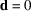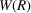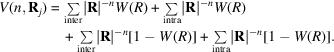International
Tables for
Crystallography
Volume B
Reciprocal space
Edited by U. Shmueli

International Tables for Crystallography (2006). Vol. B, ch. 3.4, p. 390   | 1 | 2 |

Section 3.4.10. Summation over the asymmetric unit and elimination of intramolecular energy terms

D. E. Williamsa

aDepartment of Chemistry, University of Louisville, Louisville, Kentucky 40292, USA

3.4.10. Summation over the asymmetric unit and elimination of intramolecular energy terms

| top | pdf |

Let us consider the case where the unit cell contains Z molecules which are related by Z symmetry operations, and it is desired to include only intermolecular distances in the summation. In the direct sum (1) the indices j and k will then run only over the asymmetric unit, and all terms withare eliminated. The calculated energy refers then to one molecule (or mole) rather than to one unit cell. The correction term (2) also refers to one molecule according to the range of j and k. Since the reciprocal-lattice sum refers to the entire unit cell, terms (3) and (4) need to be divided by Z to refer the energy to one molecule.

Both the direct and reciprocal sums must be corrected for the elimination of intramolecular terms. Using the convergence function, we haveAs mentioned above, the second summation term, which is the intramolecular term in direct space, is simply left out of the calculation. When using the accelerated-convergence method the third and fourth summation terms are always obtained, evaluated in reciprocal space. The undesired inclusion of the intramolecular term (fourth term above) in the reciprocal-space sum may be compensated for by explicit subtraction of this term from the sum.